# Study on the Acoustic Emission Features of Leakage Detection Assisted by Wave Guide Rods

Study on the Acoustic Emission Features of Leakage Detection Assisted by Wave Guide Rods

Wang Xinhua Jiao Yulin Niu Yongchao Yang Jie

Wuhan University of Technology, Wuhan 430063, China;

Shangqiu Vocational and Technical College, Shangqiu 476000, China;

Shangqiu Jinpeng Industrial Co.,Ltd., Shangqiu 476000,China.

Corresponding Author Email:
gyxxwxh@163.com
Page:
143-15
|
DOI:
https://doi.org/10.18280/ijht.330223
N/A
|
Accepted:
N/A
|
Published:
30 June 2015
| Citation

OPEN ACCESS

Abstract:

Through theoretical derivation of elastic waves in wave guide rods, a mathematical expression for extent calculation of acoustic emission signal propagated in the wave guide rods is constructed. The expression is tested and further studied based on which the paper proposes a method of online testing and positioning accurately the inner source of leaks in high-pressure heater by adopting measurement technology assisted by wave guide rods and source-positioning technology of acoustic emission. This method solved the leakage detection issue of high-pressure and high-temperature heater under special working conditions.

Keywords:

acoustic emission; high-pressure heater; leakage; wave guide rods; acoustic emission signal.

1. Introduction

Leakage of high-pressure heater is one of the accidents that often happen in thermal power plant. It not only affects the economical efficiency of the unit set but also causes possibly the water entering of steam turbine harming the security of equipments. High-pressure heaters, drain coolers and distillate coolers of steam turbine generator unit are connected by drain pipes. Since that while leakage occurs in one place, water levels of other places also change. So it is hard to find out accurately where the leakage point is in time[1-7].

During industrial processes, periodic detections need to be proceeded to pressure vessels, boilers and pipes with non-destructive testing technology (NDT technology) being adopted. Sensors are hard to be installed on the surface of detected target due to harsh underground testing conditions such as high temperature, copious cooling and radiation. Thus wave guide rods need to be adopted as an assisted acoustic emission detecting tool [8-13].

Wave guide rods are consisted of straight rods or those with joints. They are adopted as engineering application of active defects acoustic emission detection of pressure vessels . In the application of wave guide rods to conduct acoustic emission test, one side of the rods is connected to the tested equipment and the other side to the sensor. The elastic waves are propagated from one side that is connected to the tested equipment to the other side that is connected to the sensor and there are multiple reflections. [15-17] In the propagation through wave guide rods, the elastic waves are influenced by the structure of the rods, types of sound source (like burst sound source and continuous sound source) and positions of sound source (like rod tip and rod wall). The waves are then different in terms of waveform, range and energy. As to the variances, very few researches are conducted both at home and abroad. Consequently the usage of wave guide rods is not effective enough [18-19] .

This paper conducts a theroretical analysis to the propagation rule of elastic waves aroused by point acoutic source in wave guide rods and stuies on acoustic emission features of wave guide rods through experiments. And finally it applies this technology to the leakage detection of heater pipes.

2. Mathematic Model of Dynamic Response of Wave Guide Rods

The experimental facilities assisted by wave guide rods are mainly wave guide rods, sensor, pre-amplifier, full-digital acoustic emission detecting system, display device and warning device. The connection sequence of facilities in the detecting system is shown in Figure 1:

## 1.png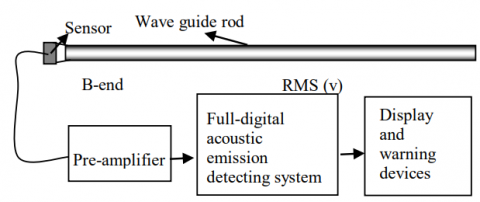Figure 1. Auxiliary detection system of waveguide bar

2.1 Mathematic model of propagation of elastic waves in wave guide rods

The waves guide rods studied belong to rod pieces. The rod length L is generally much bigger than its diameter D. When D< (5~10) L and $\lambda$>2D (here $\lambda$ only represents wave length, $\lambda=c / f$ ), wave guide rods can be simplified into one-dimensional elastic rod pieces. Thus they should be studied with one-dimensional wave theory .Suppose wave guide rods satisfy the following conditions:

a: The materials of wave guide rods are homogeneous and satisfy isotropy and comply with Hooke Law;

b: The vibration displacement is very small so that its response to power stimulation is always linearly elastic;

c: During the longitudinal vibration of wave guide rods, their cross sections are planes and the stress on every plane is homogeneously distributed.

If a point acoustic source is added to one side of wave guide rods, then there will be elastic waves in the rods. And the propagation rule of the elastic waves in the rods obeys one-dimensional wave equation which is:

$\frac{\partial^{2} u}{\partial t^{2}}=C_{0}^{2} \frac{\partial^{2} u}{\partial x^{2}}$     (1)

In the equation:

$u$——axial displacement, mm;

$x$——a random length coordinates on the rod, mm;

$T$——propagation time, s;

$C_{0}=\sqrt{\rho / E}$ , in which $\rho$——material mean density of wave guide rods, $E$——modulus.

Suppose wave guide rods receive impulse effects with transient exciting force being $I$, and then the original displacement and original speed of the rod vibration are respectively:

$\left\{\begin{array}{c}u(x, 0)=0 \\ \frac{\partial u(x, 0)}{\partial t}=\frac{I \delta(x)}{A \rho}\end{array} \quad 0 \leq x \leq L\right.$      (2)

In the equation, $\delta(x)$ is Dirac function, impulse function as well, $A$ is the cross sectional area of wave guide rods.

2.2 Mathematic model of end-part displacement of wave guide rods

Boundary conditions of wave guide rods vibration are:

$\left\{\begin{array}{l}\left.\frac{\partial u}{\partial x}\right|_{x=0}=0 \\ \left.\frac{\partial u}{\partial x}\right|_{x=L}=0\end{array}\right.$    (3)

According to (1) and (2), the mathematic expression of displacement response of wave guide rods when one side is affected by transient exciting force can be obtained:

$u(x, t)=\frac{2 I}{M} e^{-\beta} \sum_{n=1}^{\infty} \frac{1}{\omega_{n}} \sin \left(\omega_{n} t\right) \cos \left(Y_{n} x\right)=\frac{2 I}{M} \sum_{n=0}^{\infty} \frac{1}{\omega_{n}} \sin \left(\omega_{n} t\right) \cos \left(Y_{n} x\right)$   (4)

In the equation: $M=\rho A L$ . It represents the mass of wave guide rods;

$Y_{n}=n \pi \pi / L(n=1,2,3, \cdots)$ is circular wave number sequence;

$\omega_{n}$ is rod damping inherent frequency, $\omega_{n}=\sqrt{Y_{n}^{2} C_{0}^{2}-\beta^{2}}=Y_{n}^{2} C_{0}^{2}$ , in which $\beta$ is medium of wave guide rods. When exciting force is added to one side of the rods and sensor is placed at the other side, then the displacement generated at the sensor side is:

$u(L, t)=\frac{2 I}{\rho A L} \sum_{n=0}^{\infty} \frac{L}{n \pi \sqrt{E / \rho}} \sin \left(\frac{n \pi \sqrt{E / \rho}}{L} t\right) \cos (n \pi)$    (5)

2.3 Mathematic model of sensor response

In order to measure displacement, relationship between sensor response and displacement needs to be constructed. For pressure crystal sensor, when force is added homogeneously to the surface of crystal, displacement $u$ is generated and the transduction relationship is:

$U=-\tau u$  (6)

Substitute equation (5) into (6), the follow is acquired:

$U=-\tau \frac{2 I}{\rho A L} \sum_{n=0}^{\infty} \frac{L}{n \pi \sqrt{E / \rho}} \sin \left(\frac{n \pi \sqrt{E / \rho}}{L}\right) \cos (n \pi)$    (7)

In the equation:

$\tau$—time, s, generally take it as 10-8s;

$U$—voltage generated, V.

The relationship between the signal strength AMP received by sensor and voltage generated by sensor piezo-electric crystal at one side of wave guide rods is:

$A M P=20 \lg \left\{-\tau \frac{2 I}{\rho A L} \sum_{n=0}^{\infty} \frac{L}{n \pi \sqrt{E / \rho}} \sin \left(\frac{n \pi \sqrt{E / \rho}}{L}\right) \cos (n \pi)\right\}$    (8)

From equation (8) it can be seen that there is logarithmic relationship between sensor responsive extent and$\tau, I$. While materials and structure of wave guide rods are determinate, if$\tau, I$ increase, the amplitude value will also increase therewith. ($\tau$——relaxation time, $I$——transient exciting force, $L$——wave guide rods length, $\rho$——material density, $E$——Young modulus).

3. Detection Experiments of Heater Leakage Assisted by Wave Guide

Acoustic emission detection devices assisted by wave guide rod that are used to detect heater leakage are set up in the laboratory. The acoustic emission detection methods and features are studied in the lab. Experimental basis is offered to field application.

The acoustic emission detecting system and device of pipe leakage are consisted of two parts. One is acoustic emission leakage malfunction online detecting system and the other one is leakage simulation system of heater. The experimental devices are as demonstrated in Figure 2:

## 2.png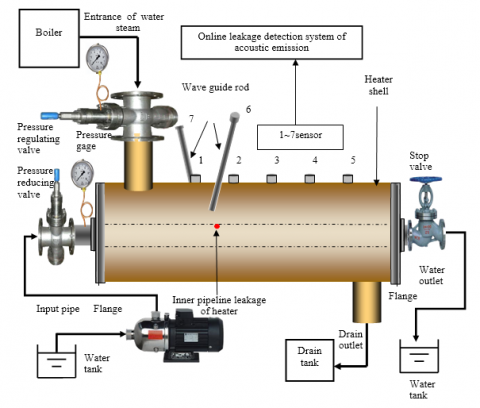Figure 2. leakage simulation system of heater assisted by wave guide rods

Two wave guide rods whose length is 500mm and diameter is 10mm are installed on heater leakage experimental devices. A hole whose diameter is 10mm is made on the outer tube wall where the No.1 wave guide rod is installed. Insert No.1 wave guide rod into the heater. The coupling between it and the inner tube wall is fine and No.1 wave guide rod is immobilized on the outer tube wall. No.2 wave guide rod is welded directly to the outer tube wall. The two wave guide rods and the sensor on the outer tube wall are on the same circular surface (make sure that two wave guide rods are on the same plane and their included angle is 90 degree; 16-channel digital acoustic emission detection system of American PAC company is adopted; WD broadband sensor with 10~1000 kHz bandwidth (PAC, USA) and 1220A pre-amplifier are adopted).

Before installation of sensor, its sensitivity is standardized. The statistical results are shown in Table 1. In Figure 2, it can be seen that sensors 1~5 are installed on the outer tube wall of the vessel. One sensor is pasted every 1 m from the leakage source. And No.6 and 7 sensors are put respectively on the two wave guide rods. No.6 is installed on the rod which is inserted into the heater and No.7 is installed on the rod which is installed directly on the outer tube wall.

Table 1. Sensor sensitivity standardized data

 sensor 1 2 3 4 5 6 7 sensitivity(dB) 92 92 91 90 90 91 90
4. Experimental Scheme

(1) Install the sensor according to the method introduced above and then connects the water inlet of heater inner pipe end and the centrifugal pump. After that pressurize and supply water to the inner pipe, gradually rising the pressure inside from 0.5～4MPa. The pressure needs to be stabilized and one set of data will be collected at the rise of every 0.5MPa. By doing so the leakage signals amplitude of the heater detector assisted by wave guide rods and the change rule of signals frequency along with inner pipe pressure will be studied.

(2) Fill the water into the heater from the steam entrance and keep the water in the heater being at quiescent state by using boiler water pump. After that pressurize and supply water to the inner pipe of the heater, gradually rising the pressure inside from 0.5～4MPa. The pressure needs to be stabilized and one set of data will be collected at the rise of every 0.5MPa. By doing so the leakage signals amplitude of the heater detector assisted by wave guide rods and the change rule of signals frequency along with inner pipe pressure will be studied.

(3) Adjust the valve of the heater dredging water gap and then pour the steam into the heater. Under regular working conditions, pressurize and supply water to the inner pipe of the heater, gradually rising the pressure inside from 0.5～4MPa. The pressure needs to be stabilized and one set of data will be collected at the rise of every 0.5MPa. By doing so the leakage signals amplitude of the heater detector assisted by wave guide rods and the change rule of signals frequency along with inner pipe pressure will be studied.

(4) Seal the leakage spot, fill the steam into the heater in a leak-tightness state. Under regular heater working conditions, collect the flow-induced noise data during the steam flow process in the heater. By doing so the leakage signals amplitude of the heater detector assisted by wave guide rods and the change rule of signals frequency along with inner pipe pressure will be studied.

(5) Change the position of intertal leakage spot and then study the change rule of leakage acoustic emission signal during the propagation through wave guide rods when the horizontal spacings between leakage spot and wave guide rods in direction of pipeline are 5cm, 50cm and 100cm respectively.

5. Experimental Data Analysis

5.1 Leakage acoustic signal features of heater

Figure 3 shows the comparative analysis of fore-and-aft oscillograms and spectrograms during the propagations of leakage acoustic emission signal through the wave guide rods under the condition of inner pressure being 0.3MPa. Figure 3(a) is the oscillograms and spectrograms of leakage acoustic emission signal of No.1 sensor at the leakage spot. Figure 3(b) and 3(c) are the oscillograms and spectrograms of leakage acoustic emission signal of No.6 and 7 sensors. From these Figure it can be seen that:

## 3a.png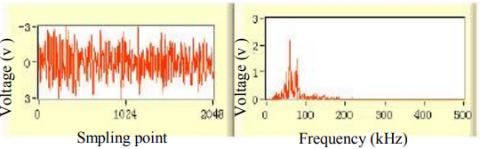(a) No.1 sensor (on the outer pipe wall)

## 3b.png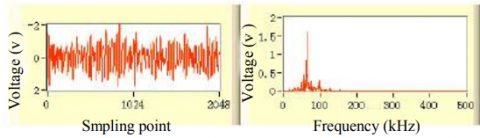(b) No.6 sensor (assisted by wave guide rods)

## 3c.png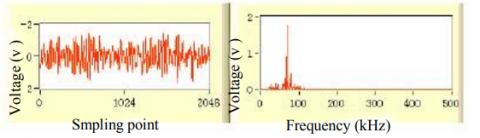(c) No.7 sensor (assisted by wave guide rods)

Figure 3. The oscillograms and spectrograms of leakage acoustic emission signal

a: After the propagation through wave guide rods, the energy of leakage acoustic emission signal declined a bit but the declining value is very small.

b: After the propagation through wave guide rods, the frequency spectrum change of leakage acoustic emission signal is not obvious. The frequency distribution range does not change at all. The main frequency elements concentrate upon 0~300 kHz.

There are no obvious differences between the acoustic emission signal range of elastic waves generated by continuous source and the leakage signals range propagated through wave guide rods. From the waveform view, the leakage signals after propagation through wave guide rods are still leakage signals and their frequency spectrum’s changes are very small. That illustrates that the changes of range, waveform and frequency spectrum of leakage acoustic emission signal after propagation through wave guide rods are small. The range decline of elastic wave propagated through wave guide rods is very small. The intrinsic waveform and frequency specturm distribution rule are maintained. Therefore, it is feasible to use wave guide rods to assist the detection under special conditions of high-temperature, low-temperature and copious cooling.

5.2 Leakage acoustic emission signal features under different pressures

Figure 4 is the changing curves of leakage signals ranges of 1~5 channels under different pressures. From the Figure it can be seen that the amplitude of leakage signals increases along with the pressure increases and declines along with the propagation distance increases. In the comparison between Figure 4(a) and 4(b), it can be seen that when there is static water in the heater, the amplitude of leakage signals declines obviously. That illustrates that the water in the heater has blocking effect on the propagation of leakage signals. And in the comparison between Figure 4(a) and 4(c), it can be seen that when there is steam flow in the heater, the amplitude of leakage signals does not decline obviously but there is obvious fluctuation of the amplitude curve. That tells that the flowing steam will generate flow-induced noise, then it has certain disturbances on the recognition of leakage signals.

## 4a.png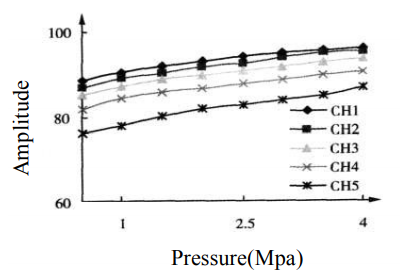(a) No steam inside the heater

## 4b.png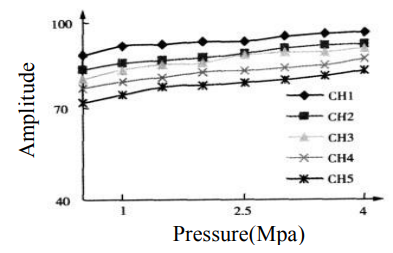(b) Static water inside the heater

## 4c.png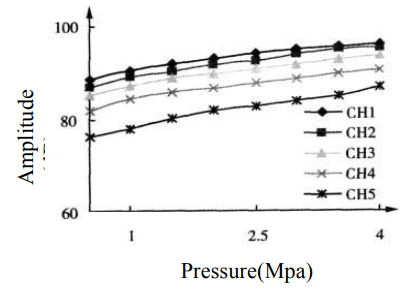(c) Flowing steam inside the heater

Figure 4. The amplitude changing curves of 1~5 channel sensor acoustic signals under different pressures

## 5a.png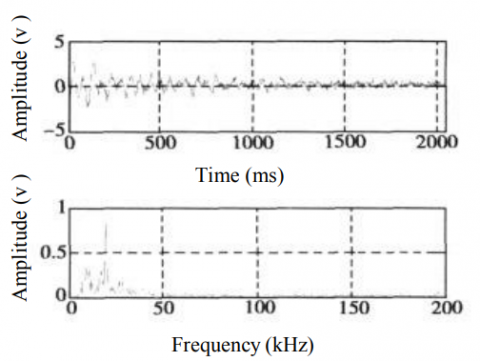(a) Heater inner steam flow-induced noise

## 5b.png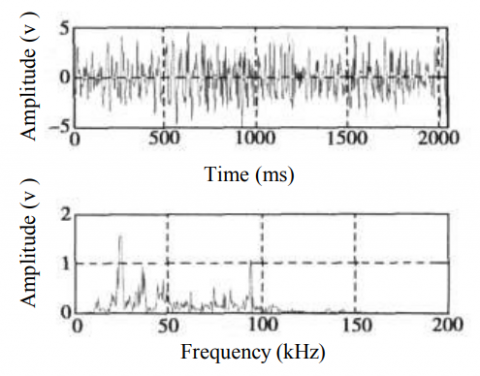(b) Leakage signals when there is no steam inside the heater

Figure 5. The oscillograms and spectrograms heater steam flow-induced noise and leakage signals

In the comparison of Figure 5(a) and (b) it can be seen that the heater steam flow-induced noise and leakage signals are continuous signals and the amplitude of flow-induced noise is relatively weaker meanwhile its time domain waveform is stable and frequency has narrow-band feature. The amplitude of leakage signals is stronger that that of flow-induced noise and its time domain waveform has bigger randomness while its frequency distribution is wider. There are many peak values within the range of 0～300 kHz.

5.3 Wavelet packet decomposition and energy distribution features at all frequency bands of leakage signals

Conduct the wavelet packet decomposition to leakage signals. From the analysis above, leakage signals frequency mainly concentrate upon 0～300 kHz. According to the sampling theorem, signal sampling frequency should be

1M

kHz, in consequence its Nyquist frequency should be 512 kHz[21-22]. Therefore decompose leakage signals to the fifth layer, there are totally 25 sub-bands. Each sub-band’s bandwidth is 16 kHz, the corresponding minimum bandwidth is 0～16 kHz.

Suppose the corresponding energy of $S_{5, j}(t)$ is $E_{5, j}$ , then:

$E_{5, j}=\left|\int S_{5, j}(t)\right|^{2} d t=\sum_{k=1}^{n}\left|x_{j, k}\right|^{2}$   (9)

In the $x_{j, k}(j=0,1,2, \cdots, n)$ test the samplings count $n=2048$. Suppose the analyzed signal’s gross energy is$E_{0}$, then:

$E_{0}=\sum_{j=0}^{2^{5}-1} E_{5, j}$   (10)

When the tested signal energy is powerful, data analysis is not easy to be conducted. Thus ratios of $E_{5, j}\left(j=0,1,2, \cdots, 2^{5}-1\right)$ and gross energy $E_{0}$ is needed to find out the energy distribution rule of leakage signals. The specific values of energy at all frequency bands and signal gross are listed in Table 2. And its two-dimensional histogram is shown in Figure 6.

Table 2. Energy distribution of signals at all frequency bands

 Number of Signal sections frequency band(kHz) The specific values of energy at all frequency bands and signal gross energy flow-induced noise leakage signals when there is no steam inside the heater leakage signals when there is static water inside the heater leakage signals when there is flowing steam inside the heater 1 0～16 0.70295 0.10113 0.26693 0.32038 2 16～32 0.21885 0.198 0.33814 0.24631 3 32～48 0.003985 0.087016 0.058192 0.1103 4 48～64 0.020652 0.24822 0.23839 0.21311 5 64～80 0.00609 0.007652 0.006287 0.004433 6 80～96 0.002599 0.037978 0.011596 0.009849 7 96～112 0.003152 0.051911 0.039155 0.058911 8 112～12 0.008321 0.22198 0.025985 0.026848 9 > 128 0.039265 0.046113 0.009859 0.015325

## 6.png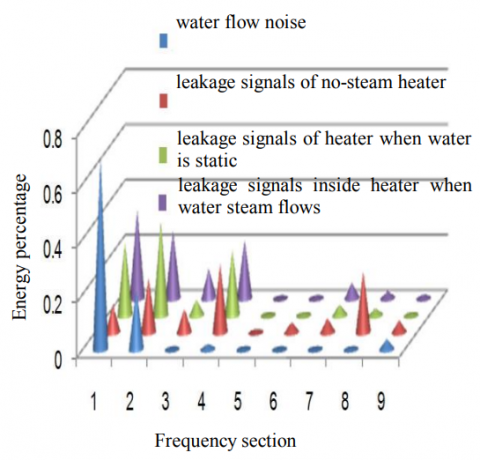Figure 6: State diagram of specific values of energy at all frequency bands and signal gross energy

a: It can be seen from Table 2 and Figure 6 that the percentage of energy with signals in between 0～128kHz in the signal gross energy are: 96.07%, 95.39%, 99.01% and 98.47% respectively. That proves that the energy of leakage signals mainly concentrate upon 0~128 kHz.

b: It can be seen from Table 2 and Figure 6 that when there is no water or steam inside the heater, the percentage of leakage signals energy in between 112~128 kHz in the signal gross energy is 22.198%. And the percentages under situations of water being static and steam flowing inside the heater are 2.5985% and 2.6848% respectively. That illustrates that water or steam inside the heater has obvious inhibition on the propagation of high-frequency leakage signals.

c: From the table 2, energy of flow-induced noise in between 0~16 kHz occupies 70.295% of the signal gross energy. And energy of leakage signals in between 0~16 kHz is less than 40% of the gross energy. The percentage of energy of leakage signals in between frequency 48~128 kHz is bigger than 30% of the leakage signals gross energy. It is much bigger than the percentage of flow-induced noise which is 3.45%. Therefore the difference between flow-induced noise and leakage signals tells whether there is leakage happen on the tubular jacketed vessel inner pipe.

5.4 Changing rule of leakage signals along with changes of propagation distance

Keep inner pressure as 0.3MPa; change the horizontal spacing between leakage spot and wave guide rods. Then extract 5 sets of data of leakage acoustic emission signals when spacing is 5cm, 50cm and 100cm respectively. Compare the characteristic parameters of leakage signals acoustic emission: peak values frequency, extent and RMS. The changing curve of leakage signals along with changes of propagation distances is as shown in Figure 7 (channel 6 is No.1 wave guide rod; channel 7 is No.2).

## 7.png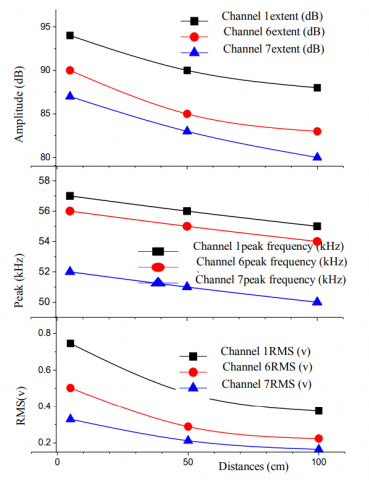Figure 7. Acoustic emission characteristic parameters of leakage signal with the propagation distance variation curve

From Figure 7:

a: No obvious changes happen to the signals extent received by sensor on wave guide rods at the same position on outer pipe wall. It is generally between 4~7dB and peak value frequency is basically the same. That tells the effect wave guide rods have on leakage acoustic signal propagation is very small. So it is feasible to apply the rods in the heater leakage test.

b: When the distance between leakage spot and wave guide rods increases, characteristic parameters will decrease therewith. However, the changing rule of each parameter remains unchanged. From this, inserting wave guide rods into the jacket or welding them on the outer pipe wall have small effect on the experimental results.

6. Conclusion

Through theoretical analysis and experimental research, it is concluded that the effects wave guide rods have on acoustic emission signal are small, so the rods can be applied in a practical test. The following conclusions are drawn from experiments:

(1) Calculation function is acquired based on the theoretical analysis and experimental verification. When the wave guide rods structure, impulse added to one end and sensor relaxation time are known, the signal amplitude received by sensor at one end can be calculated;

$A M P=20 \lg \left\{-\tau \frac{2 I}{\rho A L} \sum_{n=0}^{\infty} \frac{L}{n \pi \sqrt{E / \rho}} \sin \left(\frac{n \pi \sqrt{E / \rho}}{L}\right) \cos (n \pi)\right\}$

There exists a Logarithmic relationship between the sensor response extent and$\tau, I$.When wave guide rods material and structure are confirmed, if$\tau, I$ ncreases, the amplitude increases therewith.($\tau$——relaxation time, $I$——transient exciting force, $L$——wave guide rods length, $\rho$——material density, $E$——Young modulus).

(2) The signal waveform received by sensors on wave guide rods and outer pipe wall at the same position are similar. The amplitude attenuation is very small while the frequency distribution is basically the same. Therefore it is feasible to apply wave guide rods to the leakage detection of heater. So it is feasible to use wave guide rods to detect inner pipe leakage in the heater and use it as supplementary facility under special conditions of high-temperature, low-temperature and copious cooling.

(3) The extent of leakage acoustic emission signal increases along with pressure increase and decreases when the propagation distance increases. When there is water or water steam in the heater, the propagation of leakage signals will be hindered and the attenuation of the signals will be accelerated. When the water flows, the flow-induced noise caused will have certain interference effects on the recognition of leakage signals.

(4) The flow-induced noise has narrow-band feature, its energy mainly concentrates upon 0~16 kHz, and the randomness of leakage signals reflects the broadband features. Its energy mainly concentrates upon 48~128 kHz. Therefore, through comparison of energy at different frequency intervals of leakage signals and flow-induced noise, it can be figured out whether leakgae happens in the tubular jacketed vessel.

(5) Through comparison of waveform and frequency spectrum of leakage signals after two different installations of wave guide rods, it is concluded that there are no obvious differences between testing results. Hence in the practical engineering detection, wave guide rods can be installed directly on the outer pipe wall of heater for the convenience of detection.

Acknowledgement

Foundation item:Nature Science Foundation of Henan Science and Technology Bureau (141008)(141080); Major science and technology research plan project in henan province (142102110028);

References

1. Narenda Kumar, Rajiv Kumar.Chapter 4–Nanomedicine for Cancer Treatment [J]. Nanotechnology and Nanomaterials in the Treatment of Life-Threatening Diseases 2014, Pages 177–246.

2. Charles W. Gehrke, Robert L. Wixom, Ernst Bayer.Chapter 5 Prominent chromatographers and their research seminal concepts in chromatography/separation sciences [J]. Journal of Chromatography Library, Volume 64, 2001, Pages 99-599.

3. Guo Fuping, Li Wei, Jiang Tao. The experimental study on the leakage of pressure pipe with the aid of the guide rod. [J]. Chemical machinery, 2009, 36(4): 287～290.

4. John X.J. Zhang, Kazunori Hoshino. Chapter 6 – Mechanical Transducers: Cantilevers, Acoustic Wave Sensors, and Thermal Sensors [J]. Molecular Sensors and Nanodevices Principles, Designs and Applications in Biomedical Engineering 2014, Pages 321–414.

5. Li Shanchun, wearing a light, the peak. Propagation characteristics of acoustic emission signal in wave guide rod [J]. Journal of Daqing Petroleum Institute, 2006, 30(5): 65~68.

6. Jiang Shiliang. Effect of wave guide rod on acoustic emission signal [J]. Nondestructive detection, 2006, 28(1): 51～52.

7. Myer Ezrin. Failure Analysis and Test Procedures [J]. Plastics Failure Guide (Second Edition) 2013, Pages 321–394.

8. S. Smolentsev, F.-C. Li, N. Morley, Y. Ueki, M. Abdou, T. Sketchley. Construction and initial operation of MHD PbLi facility at UCLA [J]. Fusion Engineering and Design, Volume 88, Issue 5, June 2013, Pages 317-326.

9. Li Wei, Guo Fuping. Experimental study on acoustic emission source propagation characteristics of waveguide. [J]. Chemical machinery, 2007, 36(4): 179～183.

10. John N Coupland, Raffaella Saggin. Ultrasonic sensors forthe food industry [J]. Advances in Food and Nutrition Research, Volume 45, 2003, Pages 101-166.

11. A. Butterworth, P. Brown, O. Brunner, E. Ciapala, H. Frischholz, G. Geschonke, E. Peschardt , J. Sladen. The LEP2 superconducting RF system [J]. Nuclear Instruments and Methods in Physics Research Section A: Accelerators, Spectrometers, Detectors and Associated Equipment, Volume 587, Issues 2–3, 21 March 2008, Pages 151-177.

12. A.C. Smith, I. Verhappen. Chapter 23-Chemical Analysis: Spectroscopy [J]. Instrumentation Reference Book (Fourth Edition), 2010, Pages 341-362.

13. Smithsonian Science Information Exchange, Inc. 1- ORGANIC SOURCES OF ENERGY [J]. Summary of International Energy Research and Development Activities 1974–1976, 2013, Pages 1-1-1-90.

14. Jiang Shiliang, Li Wei. Effect of wave guide rod on acoustic emission signal. China's tenth Symposium on acoustic emission. Daqing: China's Non Destructive Testing Institute. 2004. 89～92.

15. Timothy G. Leighton. What is ultrasound?[J]. Progress in Biophysics and Molecular Biology, Volume 93, Issues 1–3, January–April 2007, Pages 3-83.

16. M. Aliofkhazraei, N. Ali.13.10-Recent Developments in Miniaturization of Sensor Technologies and Their Applications [J]. Comprehensive Materials Processing, Volume 13, 2014, Pages 245-306.

17. Mao Hanying, Huang Zhenfeng, Wei Hua, Mao Hanling. The design and experiment of the resonant conducting rod in the acoustic emission signal detection.[J]. Vibration and impact, 2014, 33(17).

18. Swapan Basu, Ajay Kumar Debnath. Chapter IVGeneral Instruments. [J]. Power Plant Instrumentation and Control Handbook, 2015, Pages 229-296.

19. Y.H. Jeong, S.S. Hwang.7-Materials management strategies for pressurized water reactors (PWRs)*[J]. Materials Ageing and Degradation in Light Water Reactors, 2013, Pages 315-334.

20. Li Wei, Guo Fuping. Experimental study on the leakage of pressure pipe with the aid of the guide rod [J]. Chemical machinery, 2009, 36(4): 287-290.

21. P. Dold, M. Heidler, A. Drevermann, G. Zimmerman.In situ observation of growth interfaces by ultrasound [J].  Journal of Crystal Growth, Volume 256, Issues 3–4, September 2003, Pages 352–360.

22. A. Kayode Coker.Chapter 18 - Compression Equipment (Including Fans) [J]. Ludwig's Applied Process Design for Chemical and Petrochemical Plants (Fourth Edition), 2015, Pages 729-978.